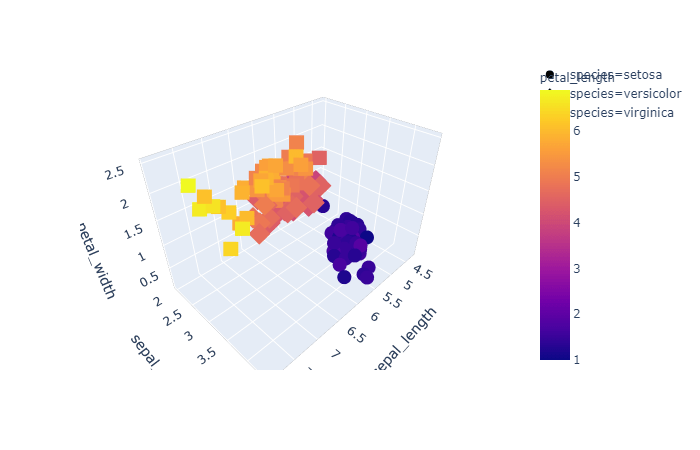# How to Create 3d Interactive Graph using Plotly

Like matplotlib and seaborn we have plotly, that is a data visualization library used for creating graphical, 3d and interactive graph. You can install plotly using below command pip install plotly Then you can import it in Jupyter notebook as below. import plotly.express as pex Let us use the famous iris dataset to create the … Continue reading How to Create 3d Interactive Graph using Plotly

# Scatter Plot using Lmplot Function of Seaborn

Apart from the methods scatterplot and regplot, seaborn also provides lmplot as another function to draw a scatterplot. However when we create scatter plots using seaborn’s lmplot, it will introduce a regression line in the plot. Let us first import libraries and load the data required to create the plot. import numpy as np import … Continue reading Scatter Plot using Lmplot Function of Seaborn

# Scatter Plot using Regplot Function of Seaborn

Though we have an obvious method named, scatterplot, provided by seaborn to draw a scatterplot, seaborn provides other methods as well to draw scatter plot. One of the other method is regplot. However when we create scatter plots using seaborn's regplot method, it will introduce a regression line in the plot as regplot is based … Continue reading Scatter Plot using Regplot Function of Seaborn# Data Visualization using Scatter Plot and Seaborn

If you have to plot your data in a graphical format smartly so that the stakeholders can understand the insight clearly and effectively then you may like to explore Seaborn. Data visualization is the presentation of data in a visual or graphical manner. With the help of data visualization, decision makers can understand difficult concepts … Continue reading Data Visualization using Scatter Plot and Seaborn